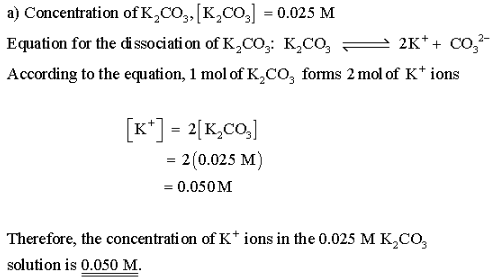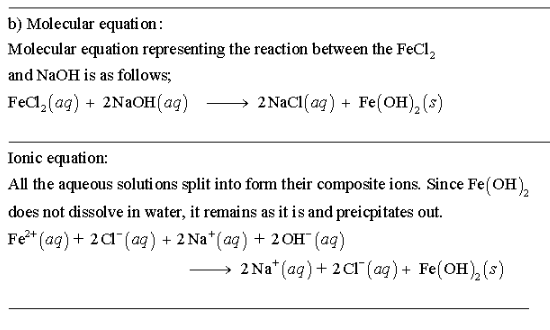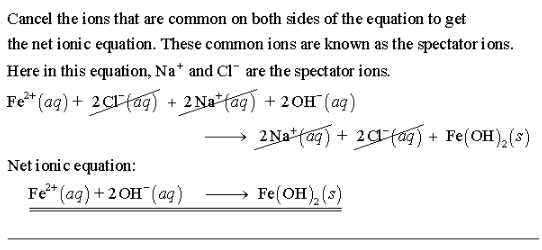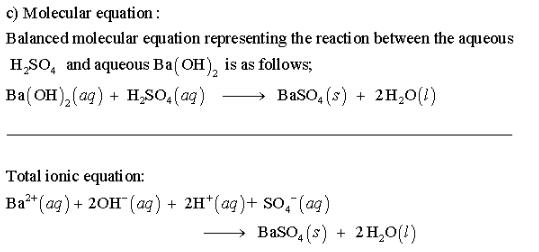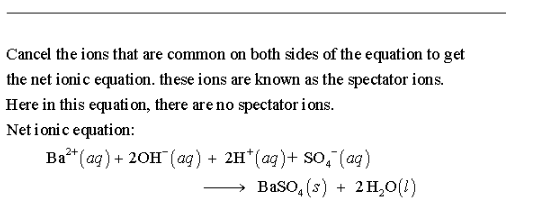# What is the concentration of K+ ions in a 0.025 K2CO3 solution assuming complete dissociation?

1.) What is the concentration of K+ ions in a 0.025 K2CO3 solution assuming complete dissociation?

2.) What is the net ionic equation of the reaction of FeCl2 with NaOH?

3.) What is the correct net ionic equation, including all coefficients, charges, and phases, for the following set of reactants? Assume that the contribution of protons from H2SO4 is near 100 %.